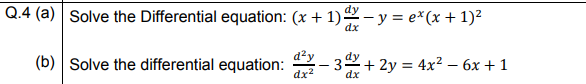# Q.4 (a) Solve the Differential equation: (x + 1) – y = e*(x + 1)² dx (b) Solve the differential equation: - 32+ 2y = 4x² – 6x + 1

Questionhelp_outlineImage TranscriptioncloseQ.4 (a) Solve the Differential equation: (x + 1) – y = e*(x + 1)² dx (b) Solve the differential equation: - 32+ 2y = 4x² – 6x + 1 fullscreen

1 Rating

### Want to see this answer and more?

Experts are waiting 24/7 to provide step-by-step solutions in as fast as 30 minutes!*

*Response times may vary by subject and question complexity. Median response time is 34 minutes for paid subscribers and may be longer for promotional offers.
Tagged in
MathCalculus

### Differential Equations